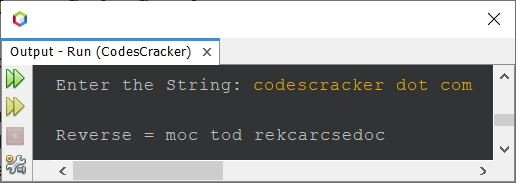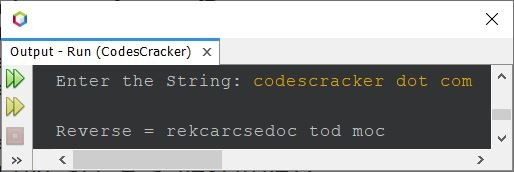# Java Program to Reverse a String

• Reverse a String using for Loop
• Reverse a String using while Loop
• Reverse a String using reverse() method
• Reverse a String using character array
• Reverse a String, word-wise

## Reverse a String in Java using for Loop

The question is, write a Java program to reverse a given string. The string must be received by user at run-time. The program given below is its answer:

```import java.util.Scanner;

public class CodesCracker
{
public static void main(String[] args)
{
String str, strReverse="";
int len, i;
char ch;
Scanner s = new Scanner(System.in);

System.out.print("Enter the String: ");
str = s.nextLine();

len = str.length();
for(i=(len-1); i>=0; i--)
{
ch = str.charAt(i);
strReverse = strReverse + ch;
}

System.out.println("\nReverse = " +strReverse);
}
}```

The snapshot given below shows the sample run of above Java program on reversing of a given string, with user input codescracker dot com as string to reverse:The string gets reversed with preserving the position of white spaces.

## Reverse a String in Java using while Loop

This program does the same job as of previous program, but created using while loop, instead of for.

```import java.util.Scanner;

public class CodesCracker
{
public static void main(String[] args)
{
String str, strReverse="";
int len, i;
char ch;
Scanner s = new Scanner(System.in);

System.out.print("Enter the String: ");
str = s.nextLine();

len = str.length();
i = (len-1);
while(i>=0)
{
ch = str.charAt(i);
strReverse = strReverse + ch;
i--;
}

System.out.println("\nReverse = " +strReverse);
}
}```

You'll get the same output as of previous program. The above program can also be created in this way too:

```import java.util.Scanner;

public class CodesCracker
{
public static void main(String[] args)
{
String strReverse="";
Scanner s = new Scanner(System.in);

System.out.print("Enter the String: ");
String str = s.nextLine();

int len = str.length();
int i = (len-1);
while(i>=0)
strReverse += str.charAt(i--);

System.out.println("\nReverse = " +strReverse);
}
}```

Still, you'll get the same output.

## Reverse a String using StringBuilder and reverse() Function

This program uses StringBuilder class and its method named reverse() to reverse a string.

```import java.util.Scanner;

public class CodesCracker
{
public static void main(String[] args)
{
Scanner s = new Scanner(System.in);

System.out.print("Enter the String: ");
String str = s.nextLine();

StringBuilder sb = new StringBuilder(str);
sb.reverse();
String strReverse = sb.toString();

System.out.println("\nReverse = " +strReverse);
}
}```

## Reverse a String in Java using a Character Array

This program uses character array to reverse a string. The toCharArray() method converts a string into character array.

```import java.util.Scanner;

public class CodesCracker
{
public static void main(String[] args)
{
String strReverse = "";
Scanner s = new Scanner(System.in);

System.out.print("Enter the String: ");
String str = s.nextLine();

char ch[] = str.toCharArray();
int len = ch.length;
for(int i=(len-1); i>=0; i--)
strReverse += ch[i];

System.out.println("\nReverse = " +strReverse);
}
}```

## Reverse a String Word Wise

This is the last program of this article. This program is little different from all the program given above. As this program reverse a string, but in word-wise manner.

```import java.util.Scanner;

public class CodesCracker
{
public static void main(String[] args)
{
String word, wordReverse;
int tot, i, len, j;
char ch;
Scanner s = new Scanner(System.in);

System.out.print("Enter the String: ");
String str = s.nextLine();

String words[] = str.split(" ");
tot = words.length;
System.out.print("\nWord Wise Reverse of String = ");
for(i=0; i<tot; i++)
{
wordReverse = "";
word = words[i];
len = word.length();
for(j=(len-1); j>=0; j--)
{
ch = word.charAt(j);
wordReverse = wordReverse + ch;
}
System.out.print(wordReverse+ " ");
}
}
}```

Here is its sample run with same user input as of first program's sample run:In above program, if user inputs a string that contains more than one white spaces in between any two or more words, then the program will not work perfectly. Therefore let me modify the program, and create a new one. This program also stores the reverse of string, word wise, then prints the whole string at last.

```import java.util.Scanner;

public class CodesCracker
{
public static void main(String[] args)
{
String strReverse = "";
String word, wordReverse;
Scanner s = new Scanner(System.in);

System.out.print("Enter the String: ");
String str = s.nextLine();

String words[] = str.split("\\s+");
int tot = words.length;

for(int i=0; i<tot; i++)
{
wordReverse = "";
word = words[i];
int len = word.length();

for(int j=(len-1); j>=0; j--)
wordReverse += word.charAt(j);

strReverse = strReverse + wordReverse + " ";
}

System.out.println("\nReverse (Word-wise) = " +strReverse);
}
}```

Here is its sample run with user input Java is Robust#### Same Program in Other Languages

Java Online Test

« Previous Program Next Program »